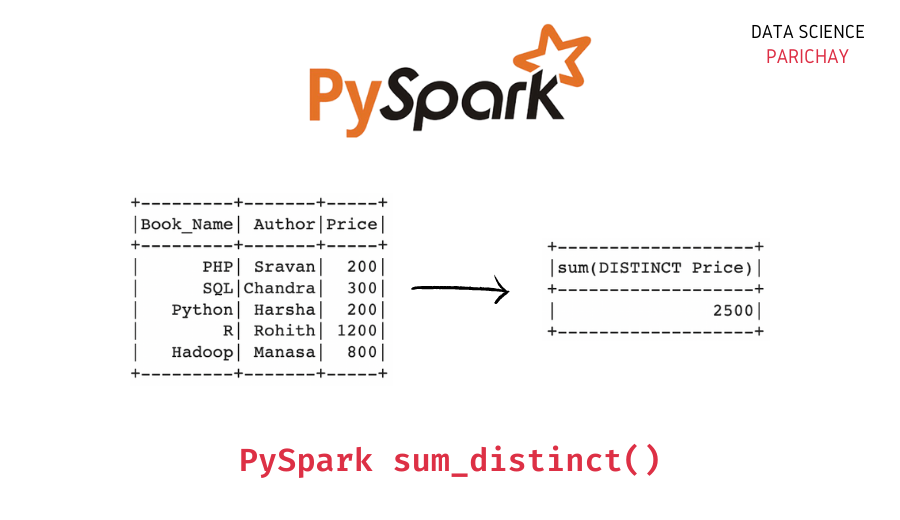# Pyspark – Sum of Distinct Values in a Column

In this tutorial, we will look at how to get the sum of the distinct values in a column of a Pyspark dataframe with the help of examples.

## How to sum unique values in a Pyspark dataframe column?

You can use the Pyspark `sum_distinct()` function to get the sum of all the distinct values in a column of a Pyspark dataframe. Pass the column name as an argument. The following is the syntax –

`sum_distinct("column")`

It returns the sum of all the unique values for the column.

## Examples

Let’s look at some examples of getting the sum of unique values in a Pyspark dataframe column. First, let’s create a Pyspark dataframe that we’ll be using throughout this tutorial.

```#import the pyspark module
import pyspark

# import the  sparksession class  from pyspark.sql
from pyspark.sql import SparkSession

# create an app from SparkSession class
spark = SparkSession.builder.appName('datascience_parichay').getOrCreate()

# books data as list of lists
df = [[1, "PHP", "Sravan", 200],
[2, "SQL", "Chandra", 300],
[3, "Python", "Harsha", 200],
[4, "R", "Rohith", 1200],
]

# creating dataframe from books data
dataframe = spark.createDataFrame(df, ['Book_Id', 'Book_Name', 'Author', 'Price'])

# display the dataframe
dataframe.show()```

Output:

```+-------+---------+-------+-----+
|Book_Id|Book_Name| Author|Price|
+-------+---------+-------+-----+
|      1|      PHP| Sravan|  200|
|      2|      SQL|Chandra|  300|
|      3|   Python| Harsha|  200|
|      4|        R| Rohith| 1200|
+-------+---------+-------+-----+```

We now have a dataframe with 5 rows and 4 columns containing information on some books.

### Sum distinct values in a column

Let’s sum the distinct values in the “Price” column. For this, use the following steps –

1. Import the `sum_distinct()` function from `pyspark.sql.functions`.
2. Use the `sum_distinct()` function along with the Pyspark dataframe `select()` function to sum the unique values in the given column.
```# import sumDistinct function
from pyspark.sql.functions import sum_distinct

# distinct value sum in the Price column
dataframe.select(sum_distinct("Price")).show()```

Output:

📚 Data Science Programs By Skill Level

Introductory

Intermediate ⭐⭐⭐

🔎 Find Data Science Programs 👨‍💻 111,889 already enrolled

Disclaimer: Data Science Parichay is reader supported. When you purchase a course through a link on this site, we may earn a small commission at no additional cost to you. Earned commissions help support this website and its team of writers.

```+-------------------+
|sum(DISTINCT Price)|
+-------------------+
|               2500|
+-------------------+```

We find the sum of unique values in the “Price” column to be 2500. This sum checks out, 200+300+1200+800=2500.

### Sum distinct values in multiple columns in Pyspark

You can also get the sum of distinct values for multiple columns in a Pyspark dataframe. Let’s sum the unique values in the “Book_Id” and the “Price” columns of the above dataframe.

```# import sumDistinct function
from pyspark.sql.functions import sum_distinct

# distinct value count in the Author and the Price columns
dataframe.select(sum_distinct("Book_Id"), sum_distinct("Price")).show()```

Output:

```+---------------------+-------------------+
|sum(DISTINCT Book_Id)|sum(DISTINCT Price)|
+---------------------+-------------------+
|                   15|               2500|
+---------------------+-------------------+```

Here, we use a `sum_distinct()` function for each column we want to compute the distinct sum of inside the `select()` function. You can see that the “Book_Id” column has a distinct value sum of 15 and the “Price” column has a distinct value sum of 2500.

You might also be interested in –

••